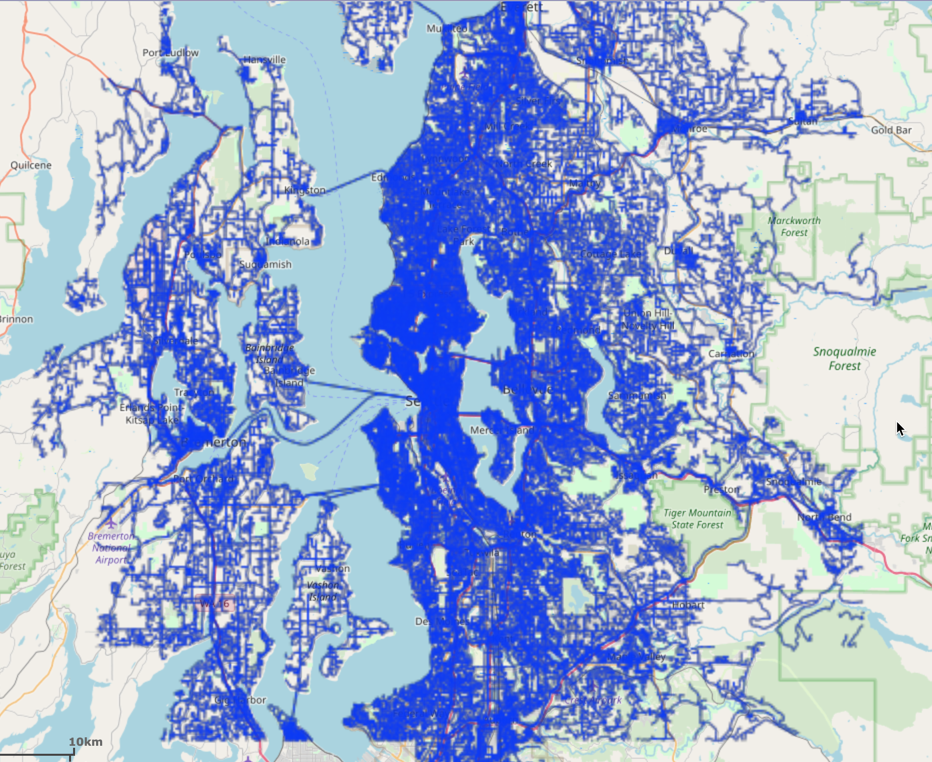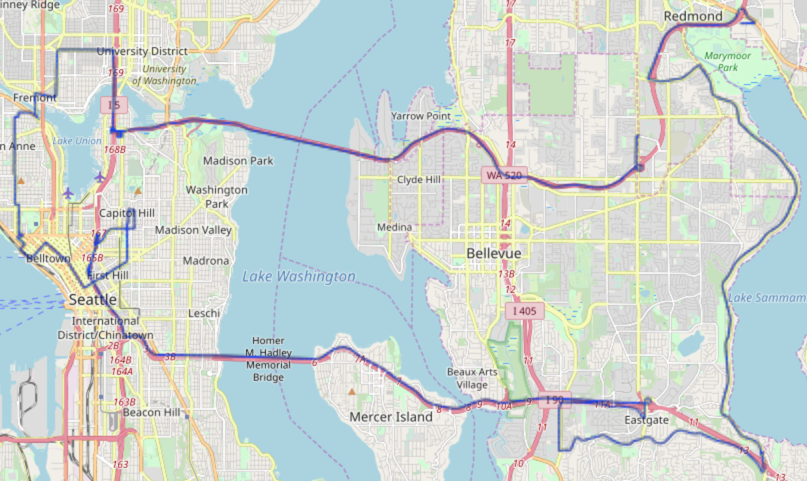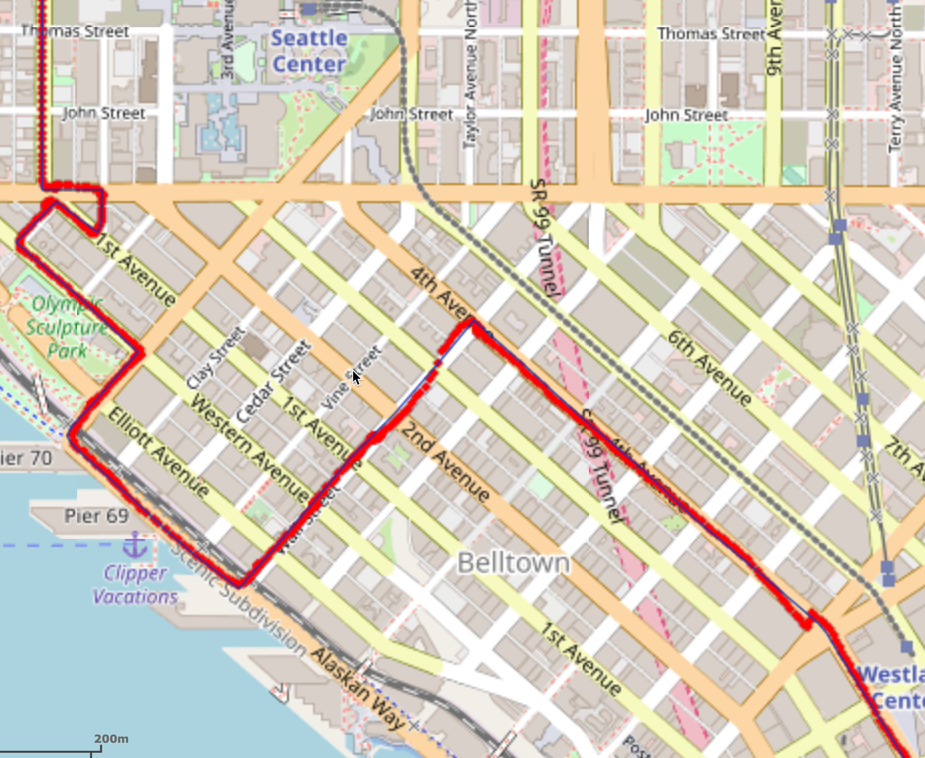# Map Matching with Python

An end-to-end example of map matching in the Python API

The following is a complete example, using the Python API, of matching GPS sample data to road network data via the /match/graph endpoint. For more information on Network Graphs & Solvers, see Network Graphs & Solvers Concepts.

## Prerequisites

The prerequisites for running the match graph example are listed below:

### Python API Installation

The native Kinetica Python API is accessible through the following means:

#### PyPI

The Python package manager, pip, is required to install the API from PyPI.

1. Install the API:

 ``````1 `````` ``````pip install gpudb --upgrade ``````
2. Test the installation:

 ``````1 `````` ``````python -c "import gpudb;print('Import Successful')" ``````

If Import Successful is displayed, the API has been installed as is ready for use.

#### Git

1. In the desired directory, run the following, but be sure to replace <kinetica-version> with the name of the installed Kinetica version, e.g., v7.1:

 ``````1 `````` ``````git clone -b release/ --single-branch https://github.com/kineticadb/kinetica-api-python.git ``````

 ``````1 `````` ``````cd kinetica-api-python ``````
3. In the root directory of the unzipped repository, install the Kinetica API:

 ``````1 `````` ``````sudo python setup.py install ``````
4. Test the installation (Python 2.7 (or greater) is necessary for running the API example):

 ``````1 `````` ``````python examples/example.py ``````

### Data Files

The example script references two data files, as mentioned in the Prerequisites, in the current local directory, by default. This directory can specified as a parameter when running the script.

## Script Detail

This example is going to demonstrate matching raw GPS points to a Seattle road network, relying on timestamps to determine the start and end point of the GPS signal.

### Constants

Several constants are defined at the beginning of the script:

• SCHEMA -- the name of the schema in which the tables supporting the graph creation and match operations will be created

Important

The schema is created during the table setup portion of the script because the schema must exist prior to creating the tables that will later support the graph creation and match operations.

• TABLE_SRN -- the name of the table into which the Seattle road network dataset is loaded

• TABLE_GPS -- the name of the table into which the raw GPS samples dataset is loaded

• TABLE_SOLUTION1 / TABLE_SOLUTION2 -- the names of the tables into which the solutions are output

• GRAPH_S -- the Seattle road network graph

Constant Definitions
 ``````1 2 3 4 5 6 7 `````` ``````SCHEMA = "graph_m_seattle_markov" TABLE_SRN = SCHEMA + ".seattle_road_network" TABLE_GPS = SCHEMA + ".raw_gps_samples" TABLE_SOLUTION1 = TABLE_SRN + "_match_solved" TABLE_SOLUTION2 = TABLE_SOLUTION1 + "_w_filter_folding" GRAPH_S = SCHEMA + ".seattle_road_network_graph" ``````

### Graph Creation

One graph is used for the match graph example utilized in the script: seattle_road_network_graph, a graph based on the road_weights dataset (one of the CSV files mentioned in Prerequisites).

The seattle_road_network_graph graph is created with the following characteristics:

• It is directed because the roads in the graph have directionality (one-way and two-way roads)
• It has no explicitly defined nodes because the example relies on implicit nodes attached to the defined edges
• The edges are identified by WKTLINE, using WKT LINESTRINGs from the WKTLINE column of the seattle_road_network table. The road segments' directionality (DIRECTION) is derived from the TwoWay column of the seattle_road_network table.
• The weights (VALUESPECIFIED) are represented using the time taken to travel the segment found in the time column of the seattle_road_network table. The weights are matched to the edges using the same WKTLINE column as edges (EDGE_WKTLINE) and the same TwoWay column as the edge direction (EDGE_DIRECTION).
• It has no inherent restrictions for any of the nodes or edges in the graph
• It will be replaced with this instance of the graph if a graph of the same name exists (recreate)
 `````` 1 2 3 4 5 6 7 8 9 10 11 12 13 14 15 16 17 18 `````` ``````create_s_graph_response = kdb.create_graph( graph_name = GRAPH_S, directed_graph = True, nodes = [], edges = [ TABLE_SRN + ".WKTLINE AS WKTLINE", TABLE_SRN + ".TwoWay AS DIRECTION" ], weights = [ TABLE_SRN + ".WKTLINE AS EDGE_WKTLINE", TABLE_SRN + ".TwoWay AS EDGE_DIRECTION", TABLE_SRN + ".time AS VALUESPECIFIED" ], restrictions = [], options = { "recreate": "true" } ) ``````

The graph output to WMS:### Matching the Graph without Fold-over Filtering

Matching to a graph typically requires another table's worth of data. In this case, the data that will be matched to the graph will come from the mm_raw_gps dataset (the other CSV file mentioned in Prerequisites). The sample points are defined using the lon and lat columns as the X and Y coordinates for each sample point; the datetime column is used for each sample point's timestamp. The time component is required for determining the start and end points of the samples.

Match Graph to GPS Data without Fold-over Filtering
 `````` 1 2 3 4 5 6 7 8 9 10 11 `````` ``````match_s_graph_response = kdb.match_graph( graph_name = GRAPH_S, sample_points = [ TABLE_GPS + ".lon AS X", TABLE_GPS + ".lat AS Y", TABLE_GPS + ".datetime AS TIME" ], solve_method = "markov_chain", solution_table = TABLE_SOLUTION1, options = {} ) ``````

The mean square error score is returned:

Match Graph to GPS Data without Fold-over Filtering Score
 ``````1 `````` ``````Score for how well the samples matched to the graph (closer to 0 is better): 0.000036783752876 ``````

The solution output to WMS:Tip

To demonstrate how successful the map matching solution was, the raw GPS samples can be overlaid on top of the solution using WMS. Below is a sample of the total solution.### Matching the Graph with Fold-over Filtering

To demonstrate how removing fold-over paths from the match solution yields a different but more accurate score, a similar /match/graph request to the above can be made but note that removing fold-over paths, e.g., setting filter_folding_paths to true, can increase execution time.

Match Graph to GPS Data with Fold-over Filtering
 `````` 1 2 3 4 5 6 7 8 9 10 11 12 13 `````` ``````match_s_graph_response = kdb.match_graph( graph_name = GRAPH_S, sample_points = [ TABLE_GPS + ".lon AS X", TABLE_GPS + ".lat AS Y", TABLE_GPS + ".datetime AS TIME" ], solve_method = "markov_chain", solution_table = TABLE_SOLUTION2, options = { "filter_folding_paths": "true" } ) ``````

The mean square error score is returned:

Match Graph to GPS Data with Fold-over Filtering Score
 ``````1 `````` ``````Score for how well the samples matched to the graph with filter folding (closer to 0 is better): 0.000037545614759 ``````

• the `match_graph_seattle_markov.py` script is in the current directory
• the `road_weights.csv` & `mm_raw_gps.csv` files are in the current directory or use the data_dir parameter to specify the local directory containing it
 ``````1 `````` ``````python match_graph_seattle_markov.py [--url ] --username --password [--data_dir ] ``````